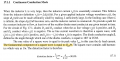# Fundamental Component of a square wave

#### Christopher B Williams

Joined Jan 9, 2021
5
Hi Guys,
Thanks for a great site.
I have read in multiple sources that the equivalent sine wave of a square wave is (4/pi) * square wave peak.
This looks correct to me, but I am trying to find mathematical proof.
Appreciate any help.

Regards
ChrisW

#### Ian0

Joined Aug 7, 2020
7,447
It rather depends on that you mean by "equivalent", but . . .
The average voltage of a sinewave is found form
$$\dfrac{\int_{0}^{\pi}\sin\theta d\theta}{\pi}$$
The integral evaluates as 2.
$$\dfrac{2}{\pi}$$ is therefore the positive-going voltage for the squarewave.
The negative half gives $$-\dfrac{2}{\pi}$$
So the peak-to-peak value is $$\dfrac{4}{\pi}$$

Last edited:

#### Christopher B Williams

Joined Jan 9, 2021
5

#### Ian0

Joined Aug 7, 2020
7,447
What you are looking at there is the Fourier transform. Put it into a graph-plotting program such as Veusz and you'll see what they mean.
Start with $$y=\dfrac{4}{\pi}\sin x$$
then try $$y=\dfrac{4}{\pi}\sin x+\dfrac{4}{\pi}\dfrac{\sin 3x}{3}$$
then try $$y=\dfrac{4}{\pi}\sin x+\dfrac{4}{\pi}\dfrac{\sin 3x}{3}+\dfrac{4}{\pi}\dfrac{\sin 5x}{5}$$

Last edited:

#### Christopher B Williams

Joined Jan 9, 2021
5
What you are looking at there is the Fourier transform.
Hi Ian,
Correct, but I am trying to get my head around where the 4/pi bit comes from.

I am reading a text book with an interest in high power rectifiers., but mainly my interest is in high power inverters for solar farms.
The ones I have been playing with are generally around 5MW made up of 3 separate inverters.

The bit that got me interested in the 4/pi thing is highlighted in yellow below:

Regards
ChrisW#### Ian0

Joined Aug 7, 2020
7,447
It comes from the Fourier integral.

#### Christopher B Williams

Joined Jan 9, 2021
5
It comes from the Fourier integral.

Thanks Ian,
I didn't have all of your message when i replied.
I will do as you suggest tomorrow. (it is getting late here, i am in Queensland Australia).
Regards
ChrisW